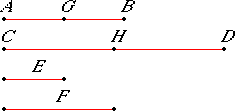# Proposition 2

If any number of magnitudes are each the same multiple of the same number of other magnitudes, then the sum is that multiple of the sum.
V.Def.2

Let any number of magnitudes AB and CD each be the same multiple of magnitudes E and F respectively.

I say that the sum of AB and CD is the same multiple of the sum of E and F that AB is of E.Since AB is the same multiple of E that CD is of F, therefore there are as many magnitudes in AB equal to E as there are in CD equal to F.

Divide AB into magnitudes AG and GB equal to E, and divide CD into CH and HD equal to F. Then the number of the magnitudes AG and GB equals the number of the magnitudes CH and HD.

Now, since AG equals E, and CH equals F, therefore the sum of AG and CH equals the sum of E and F.

For the same reason GB equals E, and the sum of GB and HD equals the sum of E and F. Therefore, there are as many magnitudes in AB equal to E as there are in the sum of AB and CD equal to the sum of E and F. Therefore, the sum of AB and CD is the same multiple of the sum of E and F that AB is of E.

Therefore, if any number of magnitudes are each the same multiple of the same number of other magnitudes, then the sum is that multiple of the sum.

Q.E.D.

## Guide

In modern terminology, this proposition states that multiplication by numbers distributes over addition of magnitudes, that is,

m (x1 + x2 + ... + xn) = m x1 + m x2 + ... + m xn.

Here, the m is a number, and all the xi’s are magnitudes of the same kind.

Euclid always displays his magnitudes as lines, but they could be magnitudes of other kinds, like plane regions, for instance. In this proposition, all the magnitudes are of the same kind.

Euclid’s proof is only for the simplest nontrivial case. He takes the number n of magnitudes to be 2, and the multiple m also to be 2, so he proves that if x1 = m y1 and x2 = m y2, then x1 + x2  = m (y1 + y2). Throughout Book V, Euclid proves the general numerical case by a particular case. The numbers he chooses are usually 2 and 3.

#### Use of this proposition

Proposition V.1 is used in the proofs of four other propositions, namely, V.5, V.8, V.12, and V.17.